# C++ Programs to Write BuzzFizz Program

In this tutorial you will learn about the C++ Programs to Write BuzzFizz Program and its application with practical example.

In this tutorial, we will learn to create a C++ program that  will find BUZZ FIZZ numbers using C++ programming.

## Prerequisites

Before starting with this tutorial we assume that you are best aware of the following C++ programming topics:

• Operators
• Basic C++ programming.
• Basic input/output.
• Looping statements.
• For Loop.
• If else statement.

## What Is Buzz Fizz?

The concept of Buzz and Fizz is that if integers value between 1 to given number,we will print “Fizz” if an integer number is divisible by 3, “Buzz” if an integer number is divisible by 5, and “FizzBuzz” if an integer number  is divisible by both 3 and 5.

## Example:

In a program that shows the numbers from 1 to number(suppose 15) .If number is a multiple of ‘3 ’ we print “Fizz” instead of if multiples of ‘5’ we print “Buzz” and if multiple of both 3 and 5 then we print “Fizz Buzz”.Following sereis will shoe Fizz anf Buzz in place or multiples.Have a look.
1, 2, Fizz, 4, Buzz, Fizz, 7, 8, Fizz, Buzz, 11, Fizz, 13, 14,
Fizz Buzz.

## Buzz Fizz Program in C++.

In this program we will print find  Buzz Fizz  using for loop. We would first declared and initialized the required variables. Next, we would prompt user to input the number of terms. Later we will  find  Buzz Fizz series of specified number of terms.

## Output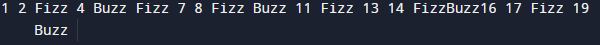In the above program, we have first declared and initialized a set variables required in the program.

• i     = for iteration of loop.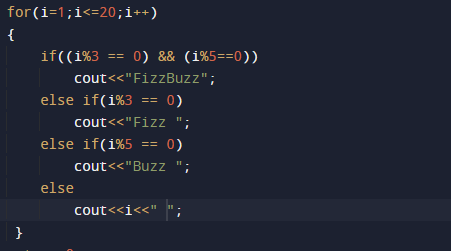In our  program we will print  number from 1 to 20. If number with in series is multiple of 3 and 5, we print “FizzBuzz” instead of the number. as you can see in image below.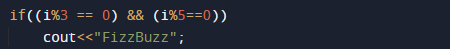Andif number is multiple of 3, print “Fizz” instead of the number.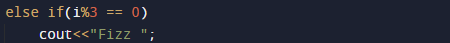And in final case if numbers which are multiples of  5, print “Buzz” instead of the number.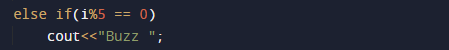And in else condition when number is not multiple of 3 and 5 we simply print the number .So in this program we  run loop from 1- 20.the final out come  series  is look like this.In this tutorial we have learn about the C++ Programs to Write BuzzFizz Program and its application with practical example. I hope you will like this tutorial.